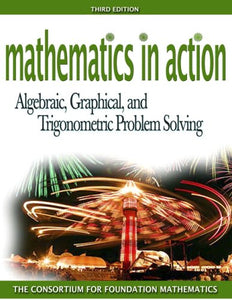# Mathematics In Action: Algebraic, Graphical, And Trigonometric Problem Solving (3Rd Edition)

• Publish Date: 2007-02-19
• Binding: Paperback
• Author: Consortium for Foundation Mathematics
• \$15.08
• Save \$112.06

Attention: For textbook, access codes and supplements are not guaranteed with used items.

Key Message: The third book of a three-part series, Algebraic, Graphics, and Trigonometric Problem Solving, Second Edition, illustrates how mathematics arises naturally from everyday situations through updated and revised real-life activities and the accompanying practice exercises. Along with the activities and the exercises within the text, MathXL and MyMathLab have been enhanced to create a better overall learning experience for the reader. Technology integrated throughout the text helps readers interpret real-life data algebraically, numerically, symbolically, and graphically. The active style of this book develops readers' mathematical literacy and builds a solid foundation for future study in mathematics and other disciplines.

Key Topics: Function Sense and Linear Functions; Introduction to Functions; Linear Functions; Systems of Linear Equations and Absolute Value Functions; The Algebra of Functions; Addition, Subtraction, and Multiplication of Functions; Composition and Inverse of Functions; Exponential and Logarithmic Functions; Exponential Functions; Logarithmic Functions; Quadratic and Higher Order Polynomial Functions; Introduction to Quadratic Functions; Complex Numbers and Problem Solving Using Quadratic Functions; Curve Fitting and Higher Order Polynomial Functions; Rational and Radical Functions; Rational Functions; Radical Functions and Equations; An Introduction to the Trigonometric Functions; Introducing the Sine, Cosine, and Tangent Functions; Why are the Trigonometric Functions Called Circular?

Market: For all readers interested in Algebra, Trigonometry.